# OECD Researchers data analysis 2 - Converting long format dataframe to wide format dataframe and merge two dataframes with RUnsplashSakuraが撮影した写真

This post is floowing of the above post.

Let's explore gdp dataframe.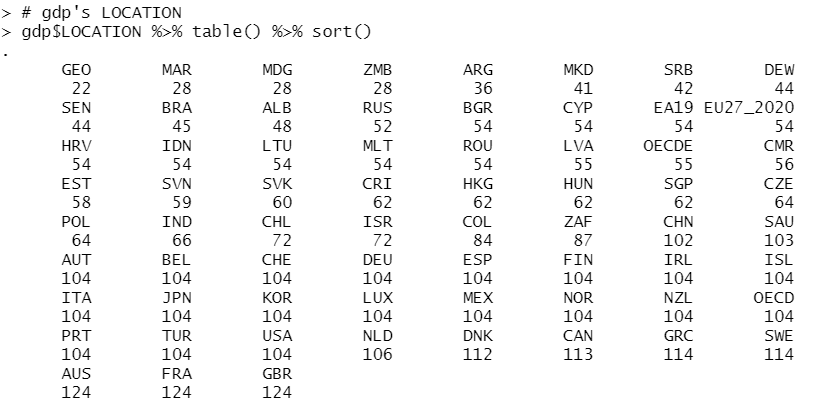gdp dataframe has more LOCATION than researcher dataframe.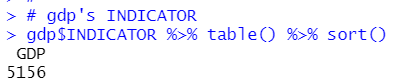gdp dataframe INDICATOR has only one calue, GDP. So I can remove it.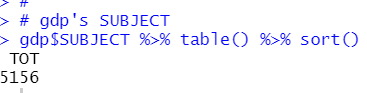gdp SUBJECT has only one value, TOT, so I can remove it.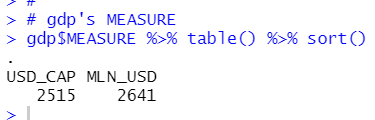gdp MEASURE has two value: USD_CAP and NLN_USD, so I cannot remove it.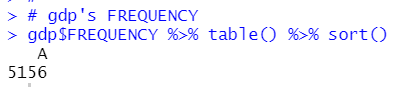gdp FREQUENCY has only one value: A. I can remove it.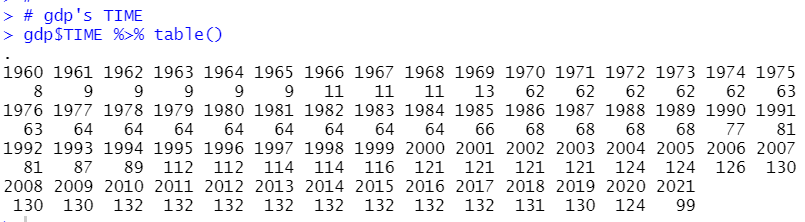gdp TIME starts at 1960 and ends at 2021.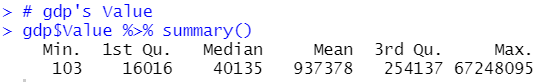So far, I can remove INDICATOR, SUBJECT and FREQUENCY from gdp dataframe.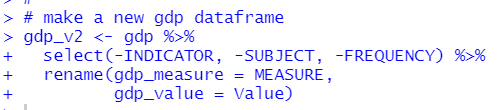Let's see new dataframe.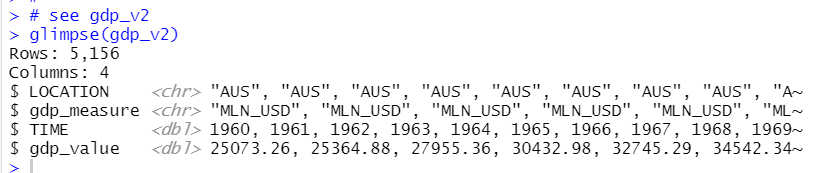Next, I merge the two dataframes, before doing that, I convert to dataframe into wide format.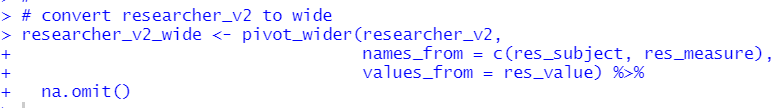Let's see this new wide format dataframe.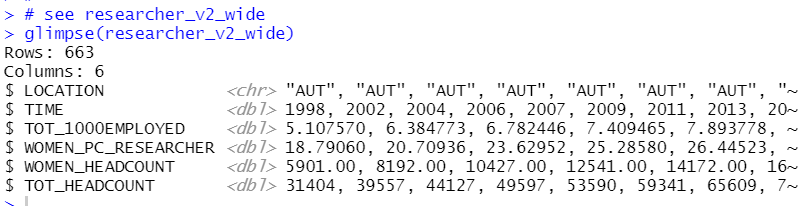I see AUT 1998 has 5.107570 for TOT_1000EMPLOYED, 18.79060 for WOMEN_PC_RESEARCHER, 5901 for WOMEN_HEADCOUNT and 31404 for TOT_HEADACOUNT.

Let's convert  gdp_v2 to wide format too.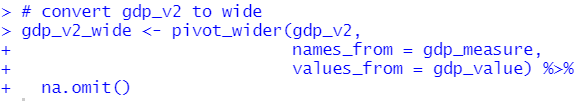Let's see it.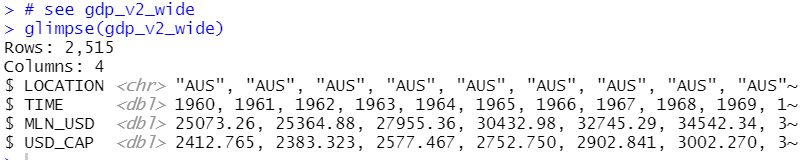AUS 1960 has 25073.26 for MLN_USD and 2412.765 for USD_CAP.

Finally, I can merge the two wide format dataframe.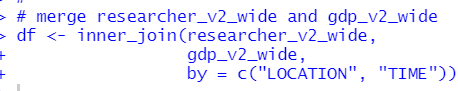Let's see it.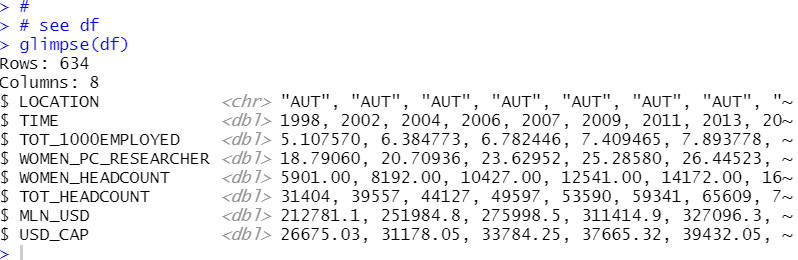Then, I convert LOCATION to factor type from character.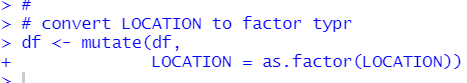Let's see summary statistics with summary() frunction.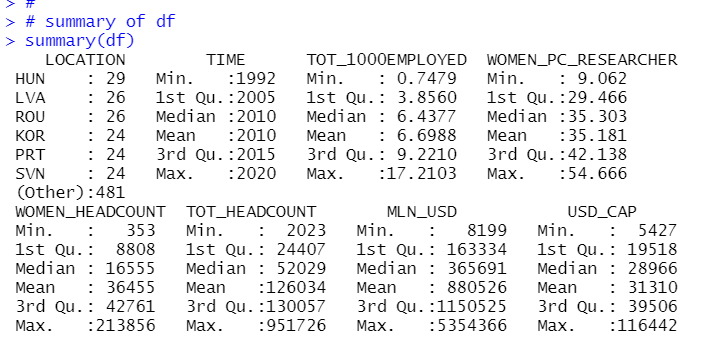I see all numeric variables are greater than zero, so I will make natural logarithm variables.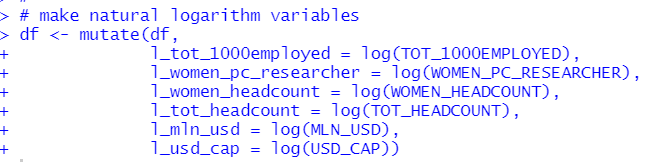Let's see log variables summary statistics.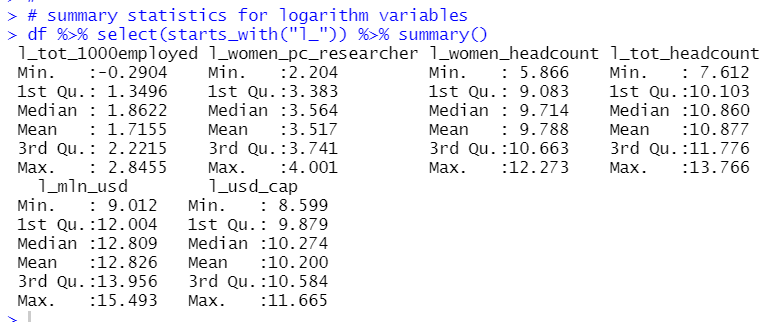Let's call it a day. Thank you!

The next post is

To read from the 1st post,